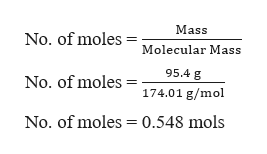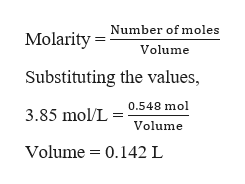# How many liters of a 3.85 M K2SO4 solution are needed to provide 95.4 g of K2SO4(molar mass 174.01 g/mol)? Recall that M is equivalent to mol/L.Express your answer to three significant figures.

Question
63 views
How many liters of a 3.85 M K2SO4 solution are needed to provide 95.4 g of K2SO4(molar mass 174.01 g/mol)? Recall that M is equivalent to mol/L.
check_circle

Step 1

The molarity of the solution is given as 3.85 M K2SO4 and it is required to calculate the volume. To calculate, first it is required to calculate the number of moles in 95.4 g of K2SO4.help_outlineImage TranscriptioncloseMass No. of moles Molecular Mass 95.4 g No. of moles 174.01 g/mol No. of moles 0.548 mols fullscreen
Step 2

Now, the molarity of the so...help_outlineImage TranscriptioncloseNumber of moles Molarity Volume Substituting the values, 0.548 mol 3.85 mol/L Volume Volume 0.142 L fullscreen

### Want to see the full answer?

See Solution

#### Want to see this answer and more?

Solutions are written by subject experts who are available 24/7. Questions are typically answered within 1 hour.*

See Solution
*Response times may vary by subject and question.
Tagged in

### General Chemistry